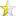Feb 3, 2009

# Multi-Dimensional Arrays

Score: 3.6/5 (86 votes)This is another topic we get asked quite a bit.
- How do I do a 2D/3D Array?

When I also started working with multi-dimensional arrays I found it hard to find the answers I wanted too. So I'll post up some info that will hopefully help other people. I'll go over both of the 2 major methods (Vector vs Pointer).

Vector based multi-dimensional arrays Vectors are a STL container that allow you to store pretty much anything in them. When used correctly they can be very powerful containers.

They provide an added benefit that they will automatically remove the memory they use when they go out of scope. This means that objects stored within a vector do not need to be de-allocated (but pointers to objects do).

You can also do some interesting things with dynamic multi-dimensional arrays with vectors. For example, if you only allocate the first dimension, then use the .push_back() to add records to the 2nd dimension it's no longer a grid, but an array with a dynamically sized 2nd dimension (much like a street of buildings each with a different amount of floors). This functionality can be achieved using pointers, but is much harder to do.

A simple 2D Array with vectors:
 ``1234567891011121314151617181920`` ``````#include using std::vector; #define HEIGHT 5 #define WIDTH 3 int main() { vector > array2D; // Set up sizes. (HEIGHT x WIDTH) array2D.resize(HEIGHT); for (int i = 0; i < HEIGHT; ++i) array2D.resize(WIDTH); // Put some values in array2D = 6.0; array2D = 5.5; return 0; }``````

A 3D Array with vectors.
 ``12345678910111213141516171819202122232425`` ``````#include using std::vector; #define HEIGHT 5 #define WIDTH 3 #define DEPTH 7 int main() { vector > > array3D; // Set up sizes. (HEIGHT x WIDTH) array3D.resize(HEIGHT); for (int i = 0; i < HEIGHT; ++i) { array3D.resize(WIDTH); for (int j = 0; j < WIDTH; ++j) array3D[j].resize(DEPTH); } // Put some values in array3D = 6.0; array3D = 5.5; return 0; }``````

Pointer based multi-dimensional arrays Pointer based multi-dimensional arrays provide you with a more raw access to the objects. The benefits can be added speed and you can apply custom optimizations to them.

Note: There are ways you can optimize this by combining the 2 dimensions into a single dimension (HEIGHTxWIDTH). I leave the discussion of this out, as it's a more advanced topic for people already familiar with this topic.

A simple 2D Array:
 ``12345678910111213141516171819202122`` ``````#define HEIGHT 5 #define WIDTH 3 int main() { double **p2DArray; // Allocate memory p2DArray = new double*[HEIGHT]; for (int i = 0; i < HEIGHT; ++i) p2DArray = new double[WIDTH]; // Assign values p2DArray = 3.6; p2DArray = 4.0; // De-Allocate memory to prevent memory leak for (int i = 0; i < HEIGHT; ++i) delete [] p2DArray; delete [] p2DArray; return 0; }``````

A 3D Array:
 ``12345678910111213141516171819202122232425262728293031`` ``````#define HEIGHT 5 #define WIDTH 3 #define DEPTH 7 int main() { double ***p2DArray; // Allocate memory p2DArray = new double**[HEIGHT]; for (int i = 0; i < HEIGHT; ++i) { p2DArray = new double*[WIDTH]; for (int j = 0; j < WIDTH; ++j) p2DArray[j] = new double[DEPTH]; } // Assign values p2DArray = 3.6; p2DArray = 4.0; // De-Allocate memory to prevent memory leak for (int i = 0; i < HEIGHT; ++i) { for (int j = 0; j < WIDTH; ++j) delete [] p2DArray[j]; delete [] p2DArray; } delete [] p2DArray; return 0; }``````

One final thing to note. When creating dynamic arrays with your own object types, you cannot overload the constructor. The ISO standard forbids this, and you must initialize the values on the objects later. All objects allocated into arrays must utilize the default constructor.

The above code snippets should compile on both Windows and Linux with no problems.

Recommendations Unless your application has an extreme need to be highly optimized, and you are quite proficient at C++ memory management, you will want to use the vector based approach. This method is a lot easier to manage, especially if you are just learning C++.
You can pass them by pointer.

e.g
`void doSomethingWith2D(double **Array);` or
`voud doSomethingWith2D(vector<vector<double> > &Array);`